# Electronics and Communication Engineering - Automatic Control Systems

### Exercise :: Automatic Control Systems - Section 2

6.

The primary function of lag compensator is to provide sufficient

 A. gain margin B. phase margin C. both gain margin and phase margin D. either (a) or (b)

Explanation:

It changes phase.

7.

In the given figure, C(s) =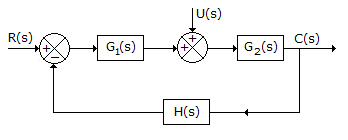A.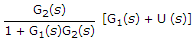B.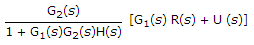C.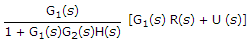D.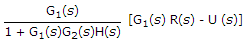Explanation:

Use principle of superposition. Initially assume R(s) = 0 and find C(s).

Then assume U(s) = 0 and find C(s). Add the two responses.

8.

For G(jω) =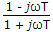A. magnitude is 1 at all frequencies and phase angle varies from 0 to - 180° as ω varies from 0 to ∞ B. magnitude is T at all frequencies and phase angle 0 C. magnitude is (1 + ω2T2)0.5 and phase angle varies from0 to 0 - 180° as ω varies from 0 to ∞ D. none of the above

Explanation: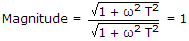When ω = 0, phase angle is 0.

For high values of phase angle = - 90° - 90° = 180°.

9.

The transient response of a second order system is given by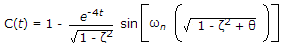for 5% criterion the settling time is

 A. 0.25 sec B. 0.75 sec C. 1.25 sec D. 4 sec

Explanation:

For 5% criterion settling time = 3T =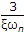.

Since ξωn = 4,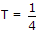and 3T = 0.75 secs.

10.

The polar plot of a transfer function passes through (-1, 0) point. The gain margin is

 A. zero B. - 1 dB C. 1 dB D. infinity